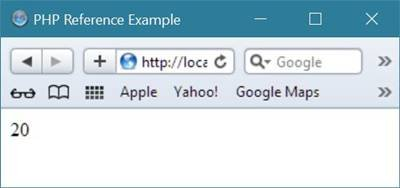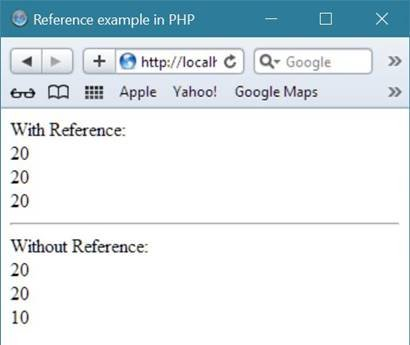# PHP Reference

Reference in PHP allows two different PHP variables to write to the same value, for example

`\$reference_var1 = &\$reference_var2;`

Here both the variable, that is, \$reference_var1 and \$reference_var2 are equal.

You can say, both variable point to the same place.

## PHP Reference Example

Let's take an example that demonstrates reference in PHP.

```<html>
<title>PHP Reference Example</title>
<body>
<?php
\$num1 = 10;
\$num2 = &\$num1;
\$sum = \$num1 + \$num2;
echo \$sum;
?>
</body>
</html>```

Below is the sample output produced by the above reference example code in PHP.## Main Use of Reference in PHP

If you assign the reference of any variable say \$first_ref_var to a another variable say \$second_ref_var, then if you change the value of variable \$first_ref_var, the \$second_ref_var's value will also be changed automatically, as you have assigned the reference of that variable.

Let's take another example to understand about the main use of reference in PHP.

```<html>
<title>Reference example in PHP</title>
<body>
<?php
echo "With Reference:<br/>";
// initialize first variable with value
\$num1 = 10;
// initialize the reference of first variable to second variable
\$num2 = &\$num1;
\$sum = \$num1 + \$num2;
echo \$sum;
echo "<br/>";
// change the value of first variable
\$num1 = 20;
echo \$num1;
echo "<br/>";
// you will see, the value of second variable will also be changed
// this is the benefit of using reference in PHP
echo \$num2;
echo "<hr/>";

echo "Without Reference:<br/>";
\$var1 = 10;
\$var2 = \$var1;
\$var1 = 20;
echo "<br/>";
echo \$var1;
echo "<br/>";
echo \$var2;
?>
</body>
</html>```

Here is the sample output of the above reference example in PHP.PHP Online Test

« Previous Tutorial Next Tutorial »